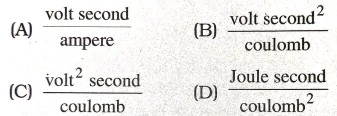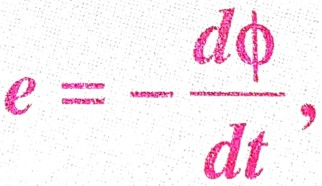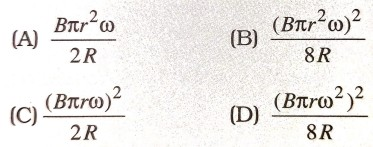1. Which of the following is not equal to a henry ?2. 1 henry is equal to :

(A) weber/ampere
(B) weber/volt
(C) weber ampere
(D) None of these

3. The magnetic field is parallel to a surface, then the magnetic flux through the surface is :

(A) zero
(B) small but not zero
(C) infinite
(D) large but not infinite

4. In the expressionthe -ve sign signifies :

(A) The induced emf is produced only when magnetic flux decreases
(B) The induced emf opposes the change in the magnetic flux.
(C) The induced emf is opposite to the direction of the flux
(D) None of these

5. The magnetic flux (Φ) linked with a coil is related to the number of turns (N) of the coil as:

(A) f α N
(B) f α N-1
(C) f α N2
(D) f α N-2

6. The magnetic flux (Φ) lined with a coil is related to its area (s) as :

(A) Φ α s
(B) Φ α s2
(C) Φ α 51/2
(D) Φ α s-1/2

7. If the magnetic flux linked with a coil through which a current of I ampere is set up is Φ, then the coefficient of self-inductance of the coil is :

(A) I/Φ
(B) Φ/I
(C) ΦI
(D) None of these

8. The role of inductance is equivalent to :

(A) inertia
(B) force
(C) energy
(D) momentum

9. In a uniform magnetic field B, a wire in the form of a semicircle of radius r rotated about the diameter of the circle with angular frequency ‘w’. The axis of rotation is perpend icular to the field. If the total resistance of the circuit is R the mean power generated per period of rotation is :